# Introducing Gelly: Graph Processing with Apache Flink

24 Aug 2015

This blog post introduces Gelly, Apache Flink’s graph-processing API and library. Flink’s native support for iterations makes it a suitable platform for large-scale graph analytics. By leveraging delta iterations, Gelly is able to map various graph processing models such as vertex-centric or gather-sum-apply to Flink dataflows.

Gelly allows Flink users to perform end-to-end data analysis in a single system. Gelly can be seamlessly used with Flink’s DataSet API, which means that pre-processing, graph creation, analysis, and post-processing can be done in the same application. At the end of this post, we will go through a step-by-step example in order to demonstrate that loading, transformation, filtering, graph creation, and analysis can be performed in a single Flink program.

Overview

## What is Gelly?

Gelly is a Graph API for Flink. It is currently supported in both Java and Scala. The Scala methods are implemented as wrappers on top of the basic Java operations. The API contains a set of utility functions for graph analysis, supports iterative graph processing and introduces a library of graph algorithms.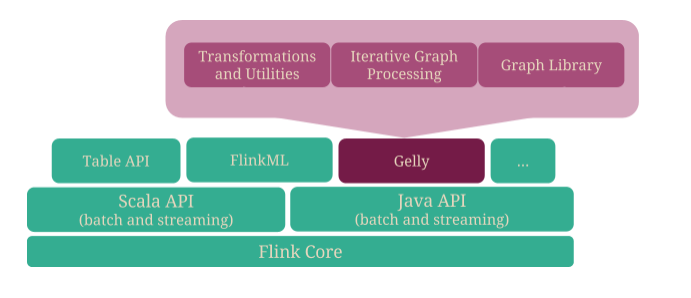## Graph Representation and Creation

In Gelly, a graph is represented by a DataSet of vertices and a DataSet of edges. A vertex is defined by its unique ID and a value, whereas an edge is defined by its source ID, target ID, and value. A vertex or edge for which a value is not specified will simply have the value type set to `NullValue`.

A graph can be created from:

1. DataSet of edges and an optional DataSet of vertices using `Graph.fromDataSet()`
2. DataSet of Tuple3 and an optional DataSet of Tuple2 using `Graph.fromTupleDataSet()`
3. Collection of edges and an optional Collection of vertices using `Graph.fromCollection()`

In all three cases, if the vertices are not provided, Gelly will automatically produce the vertex IDs from the edge source and target IDs.

## Transformations and Utilities

These are methods of the Graph class and include common graph metrics, transformations and mutations as well as neighborhood aggregations.

#### Common Graph Metrics

These methods can be used to retrieve several graph metrics and properties, such as the number of vertices, edges and the node degrees.

#### Transformations

The transformation methods enable several Graph operations, using high-level functions similar to the ones provided by the batch processing API. These transformations can be applied one after the other, yielding a new Graph after each step, in a fashion similar to operators on DataSets:

``inputGraph.getUndirected().mapEdges(new CustomEdgeMapper());``

Transformations can be applied on:

1. Vertices: `mapVertices`, `joinWithVertices`, `filterOnVertices`, `addVertex`, …
2. Edges: `mapEdges`, `filterOnEdges`, `removeEdge`, …
3. Triplets (source vertex, target vertex, edge): `getTriplets`

#### Neighborhood Aggregations

Neighborhood methods allow vertices to perform an aggregation on their first-hop neighborhood. This provides a vertex-centric view, where each vertex can access its neighboring edges and neighbor values.

`reduceOnEdges()` provides access to the neighboring edges of a vertex, i.e. the edge value and the vertex ID of the edge endpoint. In order to also access the neighboring vertices’ values, one should call the `reduceOnNeighbors()` function. The scope of the neighborhood is defined by the EdgeDirection parameter, which can be IN, OUT or ALL, to gather in-coming, out-going or all edges (neighbors) of a vertex.

The two neighborhood functions mentioned above can only be used when the aggregation function is associative and commutative. In case the function does not comply with these restrictions or if it is desirable to return zero, one or more values per vertex, the more general `groupReduceOnEdges()` and `groupReduceOnNeighbors()` functions must be called.

Consider the following graph, for instance: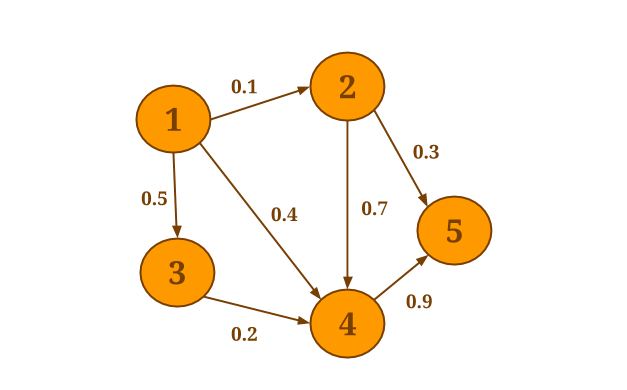Assume you would want to compute the sum of the values of all incoming neighbors for each vertex. We will call the `reduceOnNeighbors()` aggregation method since the sum is an associative and commutative operation and the neighbors’ values are needed:

``graph.reduceOnNeighbors(new SumValues(), EdgeDirection.IN);``

The vertex with id 1 is the only node that has no incoming edges. The result is therefore: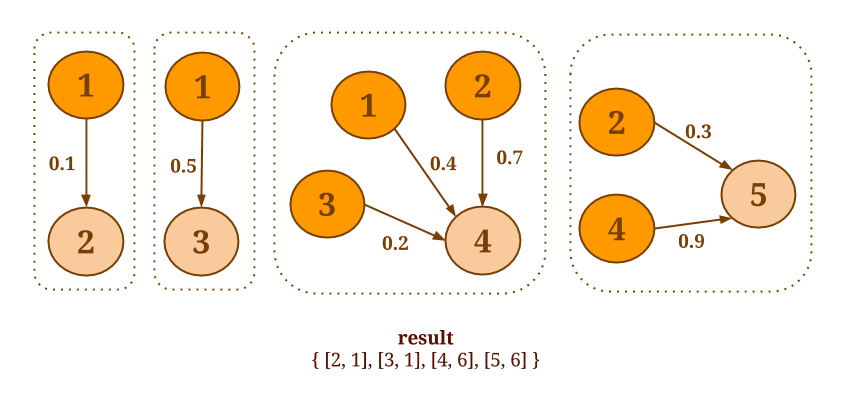## Iterative Graph Processing

During the past few years, many different programming models for distributed graph processing have been introduced: vertex-centric, partition-centric, gather-apply-scatter, edge-centric, neighborhood-centric. Each one of these models targets a specific class of graph applications and each corresponding system implementation optimizes the runtime respectively. In Gelly, we would like to exploit the flexible dataflow model and the efficient iterations of Flink, to support multiple distributed graph processing models on top of the same system.

Currently, Gelly has methods for writing vertex-centric programs and provides support for programs implemented using the gather-sum(accumulate)-apply model. We are also considering to offer support for the partition-centric computation model, using Fink’s `mapPartition()` operator. This model exposes the partition structure to the user and allows local graph structure exploitation inside a partition to avoid unnecessary communication.

#### Vertex-centric

Gelly wraps Flink’s Spargel APi to support the vertex-centric, Pregel-like programming model. Gelly’s `runVertexCentricIteration` method accepts two user-defined functions:

1. MessagingFunction: defines what messages a vertex sends out for the next superstep.
2. VertexUpdateFunction:* defines how a vertex will update its value based on the received messages.

The method will execute the vertex-centric iteration on the input Graph and return a new Graph, with updated vertex values.

Gelly’s vertex-centric programming model exploits Flink’s efficient delta iteration operators. Many iterative graph algorithms expose non-uniform behavior, where some vertices converge to their final value faster than others. In such cases, the number of vertices that need to be recomputed during an iteration decreases as the algorithm moves towards convergence.

For example, consider a Single Source Shortest Paths problem on the following graph, where S is the source node, i is the iteration counter and the edge values represent distances between nodes:In each iteration, a vertex receives distances from its neighbors and adopts the minimum of these distances and its current distance as the new value. Then, it propagates its new value to its neighbors. If a vertex does not change value during an iteration, there is no need for it to propagate its old distance to its neighbors; as they have already taken it into account.

Flink’s `IterateDelta` operator permits exploitation of this property as well as the execution of computations solely on the active parts of the graph. The operator receives two inputs:

1. the Solution Set, which represents the current state of the input and
2. the Workset, which determines which parts of the graph will be recomputed in the next iteration.

In the SSSP example above, the Workset contains the vertices which update their distances. The user-defined iterative function is applied on these inputs to produce state updates. These updates are efficiently applied on the state, which is kept in memory.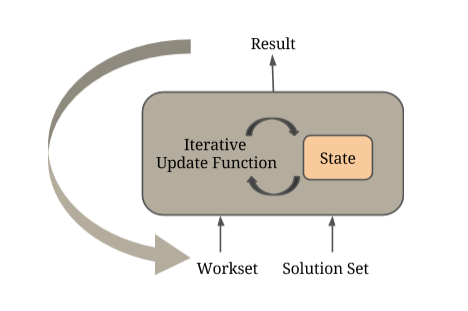Internally, a vertex-centric iteration is a Flink delta iteration, where the initial Solution Set is the vertex set of the input graph and the Workset is created by selecting the active vertices, i.e. the ones that updated their value in the previous iteration. The messaging and vertex-update functions are user-defined functions wrapped inside coGroup operators. In each superstep, the active vertices (Workset) are coGrouped with the edges to generate the neighborhoods for each vertex. The messaging function is then applied on each neighborhood. Next, the result of the messaging function is coGrouped with the current vertex values (Solution Set) and the user-defined vertex-update function is applied on the result. The output of this coGroup operator is finally used to update the Solution Set and create the Workset input for the next iteration.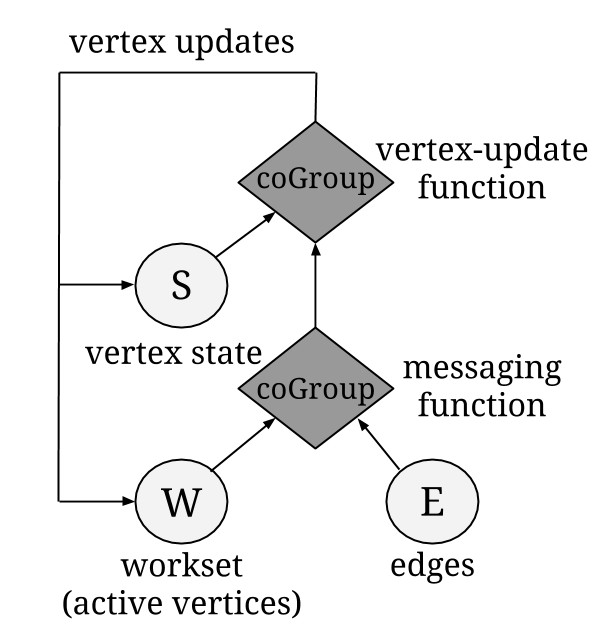#### Gather-Sum-Apply

Gelly supports a variation of the popular Gather-Sum-Apply-Scatter computation model, introduced by PowerGraph. In GSA, a vertex pulls information from its neighbors as opposed to the vertex-centric approach where the updates are pushed from the incoming neighbors. The `runGatherSumApplyIteration()` accepts three user-defined functions:

1. GatherFunction: gathers neighboring partial values along in-edges.
2. SumFunction: accumulates/reduces the values into a single one.
3. ApplyFunction: uses the result computed in the sum phase to update the current vertex’s value.

Similarly to vertex-centric, GSA leverages Flink’s delta iteration operators as, in many cases, vertex values do not need to be recomputed during an iteration.

Let us reconsider the Single Source Shortest Paths algorithm. In each iteration, a vertex:

1. Gather retrieves distances from its neighbors summed up with the corresponding edge values;
2. Sum compares the newly obtained distances in order to extract the minimum;
3. Apply and finally adopts the minimum distance computed in the sum step, provided that it is lower than its current value. If a vertex’s value does not change during an iteration, it no longer propagates its distance.

Internally, a Gather-Sum-Apply Iteration is a Flink delta iteration where the initial solution set is the vertex input set and the workset is created by selecting the active vertices.

The three functions: gather, sum and apply are user-defined functions wrapped in map, reduce and join operators respectively. In each superstep, the active vertices are joined with the edges in order to create neighborhoods for each vertex. The gather function is then applied on the neighborhood values via a map function. Afterwards, the result is grouped by the vertex ID and reduced using the sum function. Finally, the outcome of the sum phase is joined with the current vertex values (solution set), the values are updated, thus creating a new workset that serves as input for the next iteration.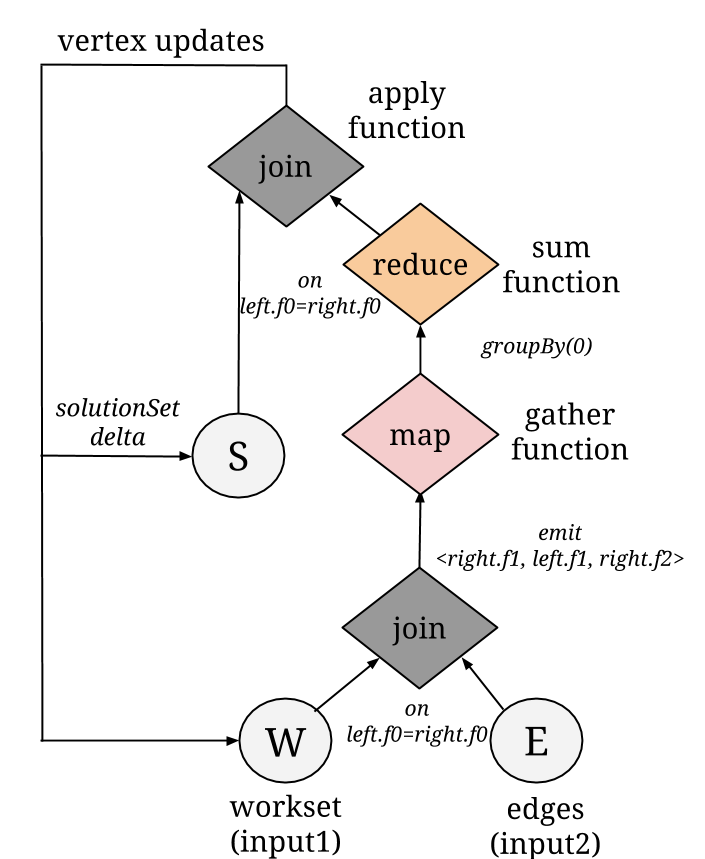## Library of Graph Algorithms

We are building a library of graph algorithms in Gelly, to easily analyze large-scale graphs. These algorithms extend the `GraphAlgorithm` interface and can be simply executed on the input graph by calling a `run()` method.

We currently have implementations of the following algorithms:

1. PageRank
2. Single-Source-Shortest-Paths
3. Label Propagation
4. Community Detection (based on this paper)
5. Connected Components
6. GSA Connected Components
7. GSA PageRank
8. GSA Single-Source-Shortest-Paths

Gelly also offers implementations of common graph algorithms through examples. Among them, one can find graph weighting schemes, like Jaccard Similarity and Euclidean Distance Weighting, as well as computation of common graph metrics.

## Use-Case: Music Profiles

In the following section, we go through a use-case scenario that combines the Flink DataSet API with Gelly in order to process users’ music preferences to suggest additions to their playlist.

First, we read a user’s music profile which is in the form of user-id, song-id and the number of plays that each song has. We then filter out the list of songs the users do not wish to see in their playlist. Then we compute the top songs per user (i.e. the songs a user listened to the most). Finally, as a separate use-case on the same data set, we create a user-user similarity graph based on the common songs and use this resulting graph to detect communities by calling Gelly’s Label Propagation library method.

For running the example implementation, please use the 0.10-SNAPSHOT version of Flink as a dependency. The full example code base can be found here. The public data set used for testing can be found here. This data set contains 48,373,586 real user-id, song-id and play-count triplets.

Note: The code snippets in this post try to reduce verbosity by skipping type parameters of generic functions. Please have a look at the full example for the correct and complete code.

After reading the `(user-id, song-id, play-count)` triplets from a CSV file and after parsing a text file in order to retrieve the list of songs that a user would not want to include in a playlist, we use a coGroup function to filter out the mismatches.

``````// read the user-song-play triplets.
DataSet<Tuple3<String, String, Integer>> triplets =
getUserSongTripletsData(env);

// read the mismatches dataset and extract the songIDs
DataSet<Tuple3<String, String, Integer>> validTriplets = triplets
.coGroup(mismatches).where(1).equalTo(0)
.with(new CoGroupFunction() {
void coGroup(Iterable triplets, Iterable invalidSongs, Collector out) {
if (!invalidSongs.iterator().hasNext()) {
for (Tuple3 triplet : triplets) { // valid triplet
out.collect(triplet);
}
}
}
}``````

The coGroup simply takes the triplets whose song-id (second field) matches the song-id from the mismatches list (first field) and if the iterator was empty for a certain triplet, meaning that there were no mismatches found, the triplet associated with that song is collected.

#### Compute the Top Songs per User

As a next step, we would like to see which songs a user played more often. To this end, we build a user-song weighted, bipartite graph in which edge source vertices are users, edge target vertices are songs and where the weight represents the number of times the user listened to that certain song.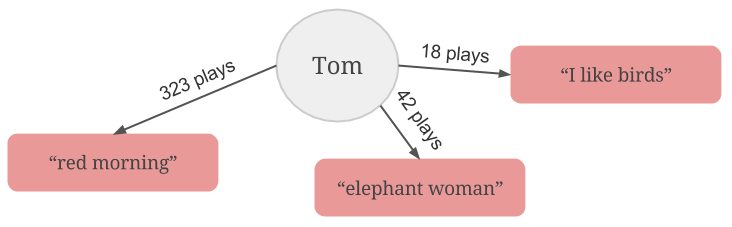``````// create a user -> song weighted bipartite graph where the edge weights
// correspond to play counts
Graph<String, NullValue, Integer> userSongGraph = Graph.fromTupleDataSet(validTriplets, env);``````

Consult the Gelly guide for guidelines on how to create a graph from a given DataSet of edges or from a collection.

To retrieve the top songs per user, we call the groupReduceOnEdges function as it perform an aggregation over the first hop neighborhood taking just the edges into consideration. We will basically iterate through the edge value and collect the target (song) of the maximum weight edge.

``````//get the top track (most listened to) for each user
DataSet<Tuple2> usersWithTopTrack = userSongGraph
.groupReduceOnEdges(new GetTopSongPerUser(), EdgeDirection.OUT);

class GetTopSongPerUser implements EdgesFunctionWithVertexValue {
void iterateEdges(Vertex vertex, Iterable<Edge> edges) {
int maxPlaycount = 0;
String topSong = "";

for (Edge edge : edges) {
if (edge.getValue() > maxPlaycount) {
maxPlaycount = edge.getValue();
topSong = edge.getTarget();
}
}
return new Tuple2(vertex.getId(), topSong);
}
}``````

#### Creating a User-User Similarity Graph

Clustering users based on common interests, in this case, common top songs, could prove to be very useful for advertisements or for recommending new musical compilations. In a user-user graph, two users who listen to the same song will simply be linked together through an edge as depicted in the figure below.To form the user-user graph in Flink, we will simply take the edges from the user-song graph (left-hand side of the image), group them by song-id, and then add all the users (source vertex ids) to an ArrayList.

We then match users who listened to the same song two by two, creating a new edge to mark their common interest (right-hand side of the image).

Afterwards, we perform a `distinct()` operation to avoid creation of duplicate data. Considering that we now have the DataSet of edges which present interest, creating a graph is as straightforward as a call to the `Graph.fromDataSet()` method.

``````// create a user-user similarity graph:
// two users that listen to the same song are connected
DataSet<Edge> similarUsers = userSongGraph.getEdges()
// filter out user-song edges that are below the playcount threshold
.filter(new FilterFunction<Edge<String, Integer>>() {
public boolean filter(Edge<String, Integer> edge) {
return (edge.getValue() > playcountThreshold);
}
})
.groupBy(1)
.reduceGroup(new GroupReduceFunction() {
void reduce(Iterable<Edge> edges, Collector<Edge> out) {
List users = new ArrayList();
for (Edge edge : edges) {
for (int i = 0; i < users.size() - 1; i++) {
for (int j = i+1; j < users.size() - 1; j++) {
out.collect(new Edge(users.get(i), users.get(j)));
}
}
}
}
})
.distinct();

Graph similarUsersGraph = Graph.fromDataSet(similarUsers).getUndirected();``````

After having created a user-user graph, it would make sense to detect the various communities formed. To do so, we first initialize each vertex with a numeric label using the `joinWithVertices()` function that takes a data set of Tuple2 as a parameter and joins the id of a vertex with the first element of the tuple, afterwards applying a map function. Finally, we call the `run()` method with the LabelPropagation library method passed as a parameter. In the end, the vertices will be updated to contain the most frequent label among their neighbors.

``````// detect user communities using label propagation
// initialize each vertex with a unique numeric label
DataSet<Tuple2<String, Long>> idsWithInitialLabels = DataSetUtils
.zipWithUniqueId(similarUsersGraph.getVertexIds())
.map(new MapFunction<Tuple2<Long, String>, Tuple2<String, Long>>() {
@Override
public Tuple2<String, Long> map(Tuple2<Long, String> tuple2) throws Exception {
return new Tuple2<String, Long>(tuple2.f1, tuple2.f0);
}
});

// update the vertex values and run the label propagation algorithm
DataSet<Vertex> verticesWithCommunity = similarUsersGraph
.joinWithVertices(idsWithlLabels, new MapFunction() {
public Long map(Tuple2 idWithLabel) {
return idWithLabel.f1;
}
})
.run(new LabelPropagation(numIterations))
.getVertices();``````

## Ongoing and Future Work

Currently, Gelly matches the basic functionalities provided by most state-of-the-art graph processing systems. Our vision is to turn Gelly into more than “yet another library for running PageRank-like algorithms” by supporting generic iterations, implementing graph partitioning, providing bipartite graph support and by offering numerous other features.

We are also enriching Flink Gelly with a set of operators suitable for highly skewed graphs as well as a Graph API built on Flink Streaming.

In the near future, we would like to see how Gelly can be integrated with graph visualization tools, graph database systems and sampling techniques.